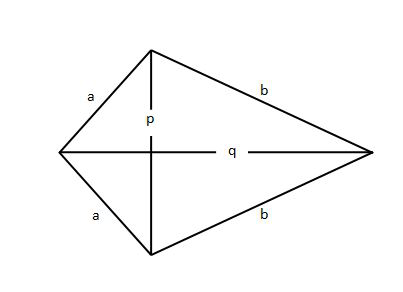# Perimeter of a Kite Formula

• Last Updated : 18 Jan, 2022

Kite is a quadrilateral in which two pairs of sides are equal and these equal sides are adjacent to each other. It is known as a parallelogram, it also has two pairs of equal sides but in a parallelogram, those pairs are opposite to one another while in the case of kite these equal pairs of sides are adjacent.Kite

In the above figure, two adjacent sides are equal so the length of both is a and the length of the other two equal sides are b.

p and q are the diagonals of the Kite.

Properties of Kite

• Kite has four sides and its geometry is similar to the quadrilateral type.
• It has four angles and four vertices.
• It has a single line of symmetry.
• Diagonals of a kite intersect at right angles.
• The length of diagonals of a kite is not equal.
• Rotational symmetry is of order 1.
• Each angle formed by adjacent unequal sides is equal.
• Each angle formed by adjacent equal sides is unequal.

Perimeter of a Kite Formula

Perimeter is the sum of all sides of a closed polygon. So to calculate the perimeter of a kite, the length of all the sides are required, or we can say only two unequal sides are required, lets call these two unequal sides a and b.

Perimeter of Kite = Sum of all sides = a+a+b+b = 2 × (a + b)

### Sample Questions

Question 1: Find the perimeter of a kite whose sides are 4 cm, 4 cm, 6 cm, 6 cm?

Perimeter of Kite = sum of all sides

= 4 + 4 + 6 + 6

= 20 cm

Question 2: Find the perimeter of a kite whose unequal sides are 8 cm and 12 cm?

Given a = 8 cm and b = 12 cm.

Perimeter of Kite = 2 × (a+b)

= 2 × (8+12)

= 40 cm

Question 3: Perimeter of a kite is 50 cm, and a side length is 4 cm find the length of other unequal side?

Given perimeter = 50 cm, lets take 4 cm as a

We need to find b.

using formula of perimeter of kite = 2×(a+b)

50 = 2×(4 + b)

50 = 8 + 2×b

×b = 50 – 8

b = 42/2

b = 21 cm

Hence the length of other unequal side is 21 cm.

Question 4: If one side of a kite is twice the other unequal side and the perimeter is 99 cm, find both the unequal sides?

Let take first unequal side is A,

then according to question A = 2×B

so B = A/2

perimeter of kite = 2×(A+B)

102  = 2×(A+A/2)                  as B = A/2

102  = 2×((3×A)/2)

102  = 3×A

A = 102/3

A = 34 cm

B = A/2 = 34/2 = 17 cm

Hence unequal sides are of length 17 cm and 34 cm.

Question 5: Perimeter of a kite is 100 cm, and the length of one side is 42 cm find the length of another unequal side?

Given perimeter = 100 cm, lets take 42 cm  as a

we need to find b.

using formula of perimeter of kite = 2×(a+b)

100  = 2×(42 + b)

100  = 84 + 2×b

2×b = 100 – 84

b = 16/2

b = 8 cm

Hence the length of other unequal side is 8 cm.

My Personal Notes arrow_drop_up